Nanomedicine, Volume I: Basic Capabilities

Robert A. Freitas Jr., Nanomedicine, Volume I: Basic Capabilities, Landes Bioscience, Georgetown, TX, 1999

9.2.1 Van der Waals Adhesion Forces

In dry or vacuum environments, adhesion forces between <100 micron diameter particles and surfaces (nanorobot and other) separated by 100 nm or less are usually dominated by van der Waals interactions.1149 Consider a spherical particle of radius r lying a small distance zsep <~ 100 nm from a flat plate of surface area A >> p r2. As a crude approximation, ignoring retardation effects that may become important in solution for zsep > 5 nm,10 the van der Waals adhesive force is approximated by:1146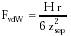{Eqn. 9.1}

for zsep << r, where H is the Hamaker constant for the interaction. (Eqn. 9.1 also applies to two crossed cylinders of equal radius.1152) The Hamaker constants for the materials comprising the sphere, the plate, and the surrounding medium are Hs, Hp, and Hm, respectively (Table 9.1), giving:1149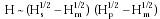{Eqn. 9.2}

The adhesion separation distance zsep is often taken as ~0.4 nm for particles in intimate (but chemically unbonded) contact with a surface.1146,1148 Hence the force required to overcome the van der Waals adhesive attraction of a perfectly rigid r = 0.5 micron particle adhered to a diamond plate in vacuo (with zsep = 0.4 nm, H = 340 zJ) is FvdW ~ 180 nN.

Real particles are not perfectly rigid but deform under the influence of this adhesive force. If the adhesion surface area is increased from point contact to a circle of radius radhesion, then the van der Waals adhesion force increases to:1146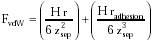{Eqn. 9.3}

According to the JKR theory of adhesion mechanics1155 for a sphere on a flat surface of the same material with a work of adhesion Wadhesion (joined surface energy; Section 9.2.3) and elastic modulus Ke:1149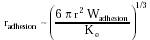{Eqn. 9.4}

Assuming Wadhesion ~10 J/m2 and Ke ~ 1012 N/m2 for smooth flawless diamond and r = 0.5 micron gives radhesion ~ 36 nm. Taking zsep = 0.4 nm contact with a flat diamond plate over a ~4100 nm2 circular adhesion spot in vacuo, then FvdW ~ 1300 nN, a mean surface pressure of pmean = FvdW / p radhesion2 ~ 3200 atm over the contact circle and a peak pressure ppeak = 1.5 pmean = 4800 atm at the center. As the sphere is pulled away, separation occurs abruptly when the contact radius falls to radhesion / 41/3 = (~63%) radhesion = 23 nm.

Van der Waals forces also depend upon the roughness of the surface. If a smooth sphere approaches a surface having uniform rugosity or roughness brug with roughness feature size << r, then the van der Waals force is approximately:1148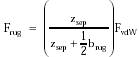{Eqn. 9.5}

For zsep = 0.4 nm and brug = 10 nm, Frug = 2% FvdW ~ 4 nN for the rigid sphere (in vacuo) in the examples given above. Surface dimpling is a common practice in MEMS fabrication, for example, to reduce adhesion between polysilicon layers and the substrate.1382

A few other geometries of rigid bodies approaching contact are useful as well. For example, the van der Waals adhesive force between two flat plates of equal interfacial area A and uniform separation zsep is:1152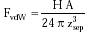{Eqn. 9.6}

Two (1 nm)2 diamond plates separated by zsep = 0.4 nm in vacuo require FvdW ~ 0.07 nN (~7 atm) to overcome the van der Waals attractive force. Such plates are the same size as the contacting end surfaces of the logic rods employed in Drexler's mechanical computer design (Section 10.2.1); the design assumes a rod realignment force of 1 nN in the exemplar calculations.10 This result suggests that forces on the order of 10-100 pN must be applied to mobile nanoscale components inside mechanical nanodevices in order to overcome static van der Waals adhesion that arises due to the contact proximity of parts within the structure.

For another example, two rigid spheres of radii r1 and r2, separated by a distance zsep << r1, r2, have a van der Waals adhesive force of:1152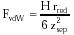{Eqn. 9.7}

where the reduced radius rred = (r1 r2) / (r1 + r2). A pair of 1-micron diameter rigid diamond spheres separated by zsep = 0.4 nm in vacuo have a van der Waals mutual adhesive force FvdW ~ 90 nN.

As one final example, the van der Waals force between two parallel cylinders of length L, radii r1 and r2, and separation zsep is (differentiating VvdW in Drexler10):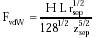{Eqn. 9.8}

A pair of 1-nm diameter, 10-nm long rigid diamondoid cylinders lying exactly zsep = 0.4 nm apart along their entire length in vacuo feel a van der Waals force of FvdW ~ 1.5 nN. In all three examples, as before, the force rises slightly if the objects deform and declines significantly if surface rugosity is increased. (See also entropic packing, Section 9.4.2.3.)

Last updated on 20 February 2003